# Microstrip Via Hole Inductance

Here we will provide an equation that allows you to calculate the inductance of a single ground via in a microstrip circuit board.

This is based on the IEEE paper "Modeling Via Grounds in Microstrip" IEEE Microwave and Guided Wave Letters, Vol. 1, No. 6, June 1991, by Goldfarb and Pucel. It is Equation 3 in the reference.

Note that this is an empirical solution that was found to fit data where the height was between 100 um and 631 microns. It is supposed to be valid for heights less that 3% of a wavelength. Note: equation graphic was corrected March 2016, the units are Henries, if the dimensions you enter are in meters. Equation graphic was further corrected in July 2017, we apologize if you had troube with it.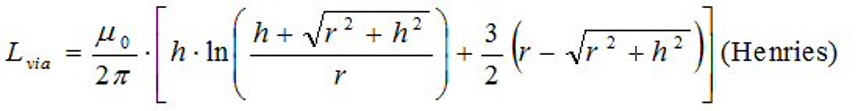Although Goldfarb and Pucel didn't tell you, the units of all of the lengths have to be in meters, then the answer comes out in Henries. We entered the equation into a spreadsheet. Here is the inductance for a via hole in 15, 10, 4 and 2 mil substrates: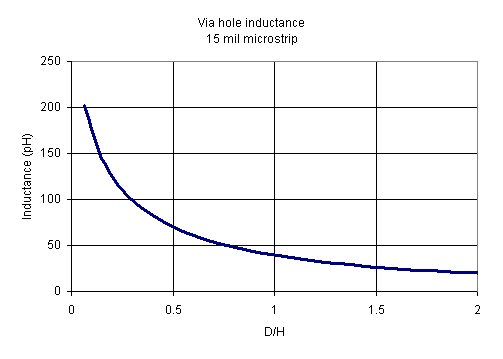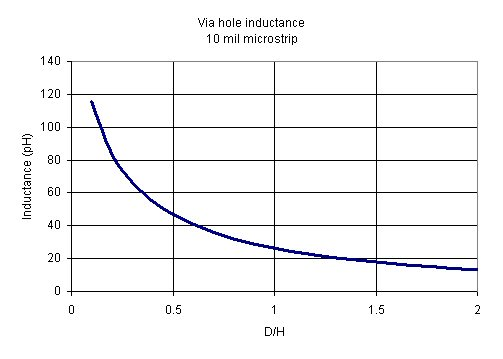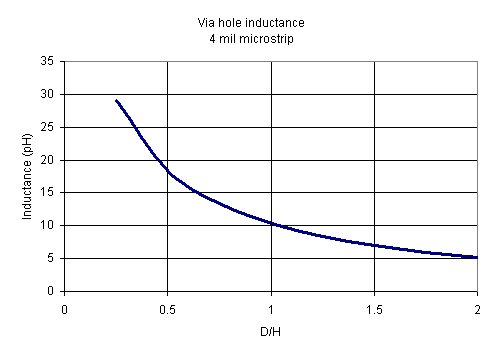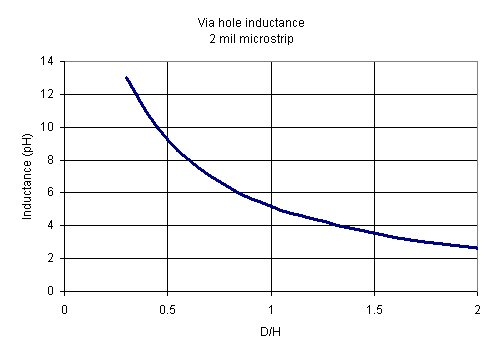In many cases it is desirable to keep the D/H ratio to 0.5 to minimize the catch-pad area (especially on MMICs).

### Via hole inductance rule of thumb(s)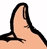On two-mil (50 micron) GaAs, Lvia is about 10 pico-henries. For four-mil (100 um) GaAs, it is about 20 pico-Henries. Note that these rules are most accurate for D/H aspect ratio of about 0.5.

### References

"Modeling Via Grounds in Microstrip" IEEE Microwave and Guided Wave Letters, Vol. 1, No. 6, June 1991, by Goldfarb and Pucel.

Author : Unknown Editor